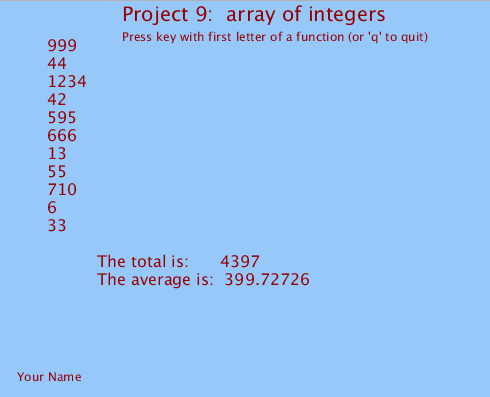## Array of integers

Create an array of integers, and display them in a list.

Write a function to add up all of the numbers in an array and return the total.
Display the sum total and mean average.NOTE:   Mean average is total divided by size of array. Since this could be a fraction, be sure to make it a "float".

### Add some functions to do the following:

big( int a[], int m )
// Move biggest number to end of the array.
// (Swap array elements, to keep all numbers.)

small( int b[], int m )
// Move smallest to beginning of array
// (Swap, to keep all numbers.)

inc( int d[], int n )
// Increase each of the numbers by one.

dec( int a[], int m )
// Decrease each of the numbers by one.

triple( int a[], int m )
// Triple each of the numbers.

randomize( int p[], int k )
// Replace all numbers of random values.
NOTE:    Do not use global values in these functions; use the arguments.

Write code in keyPressed()
to call each function when a key is pressed, with the first letter of that function.
 ``` b big() // Move biggest to end of array. s small() // Move smallest to beginning. i inc() // Increase each by one. d dec() // Decrease each by one. t triple() // Triple each ofthe numbers. r randomize() // Replace all with random values. ```

BUTTONS
create buttons with the names these mwthods,
and add mousePressed() code to call them when button is pressed.